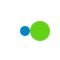##### 征服宝石 (Gem of Conquest)圣痕\武器 木剑 金剑 石剑 铁剑 钻石剑 天殛之钥 无 3+3+3+6+6（21） 3+3+3+6+6（21） 3+3+3+6+6（21） 3+3+3+6+6（21） 3+3+3+6+6（21） 4+5+4+9+9（31） 贝纳勒斯·觉醒上 3+4+3+7+8（25） 3+4+3+7+8（25） 3+4+3+7+8（25） 3+4+3+7+8（25） 3+4+3+7+8（25） 5+5+6+11+10（37） 贝纳勒斯·觉醒中 3+4+4+7+8（26） 3+4+4+7+8（26） 3+4+4+7+8（26） 3+4+4+7+8（26） 3+4+4+7+8（26） 5+5+5+11+10（36） 贝纳勒斯·觉醒下 3+4+4+7+8（26） 3+4+4+7+8（26） 3+4+4+7+8（26） 3+4+4+7+8（26） 3+4+4+7+8（26） 5+5+5+11+10（36） 贝纳勒斯·觉醒上中 4+5+4+9+9（33） 4+5+4+9+9（33） 4+5+4+9+9（33） 4+5+4+9+9（33） 4+5+4+9+9（33） 6+6+6+13+13（44） 贝纳勒斯·觉醒上下 4+5+4+9+9（33） 4+5+4+9+9（33） 4+5+4+9+9（33） 4+5+4+9+9（33） 4+5+4+9+9（33） 6+6+6+13+13（44） 贝纳勒斯·觉醒中下 4+5+4+9+9（31） 4+5+4+9+9（31） 4+5+4+9+9（31） 4+5+4+9+9（31） 4+5+4+9+9（31） 6+6+6+12+12（42） 贝纳勒斯·觉醒上中下 5+5+6+11+10（37） 5+5+6+11+10（37） 5+5+6+11+10（37） 5+5+6+11+10（37） 5+5+6+11+10（37） 7+7+7+14+15（50）